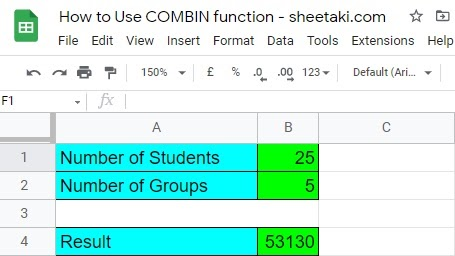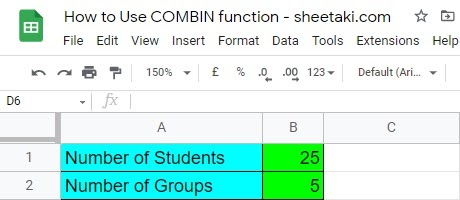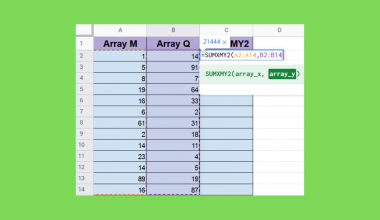How to Use COMBIN Function in Google Sheets

The COMBIN function in Google Sheets helps you quickly calculate the estimated number of groups for a specific number of items.

The rules for using the COMBIN function in Google Sheets are as follows:

• The function only requires two arguments to determine the estimated number of groups.
• The COMBIN function only works with integer values.
• It does not work in finding repetitive data.
• The value of each argument should be more than or equal to “0”. Otherwise, a “#NUM!” error message will appear.

Let’s see how the COMBIN function in Google Sheets works in the example below.

A professor wanted to divide her students according to the number of students required per reporting task. She needs to group her 25 students into 5 groups.

The professor can now easily pull up the estimated number of total combinations with the given data. However, to quickly generate the answer, she must use the COMBIN function in Google Sheets to see the number of times to produce a  5-person-team from the whole group.

Based on the given, the result yielded by the Google Sheets’COMBIN function is around 53,130 possible combinations.

Now that we have tried to apply the COMBIN function in a real-life scenario, let us begin understanding the formula’s parts and practice writing it to understand how it works fully.

Here’s another example of how the COMBIN function helps you quickly find the total number of groups in specific data.

For example, you are given a task to solve a problem and you need to determine the number of combinations from the data below. In addition to that, you must not have any combinations or data repeated when solving the problem.

 Set Pool of Numbers to Choose From Numbers of Objects to Pick 1 5 5 2 10 5 3 15 5 4 20 5 5 25 5 6 30 5

Because you already have an overview of how to use the COMBIN function, you can easily generate all the answers from the data above.

To get the exact result, we need to substitute the Google Sheet’s COMBIN function’s formula with the real data.

=COMBIN(n, k)

Where:

n=Pool of Numbers to Choose From

k=Numbers of objects to pick

After substituting the data, here’s how it looks now.The results on the far right show the total number of combinations without repetitions.

If you are wondering how it was done, let us check the fundamentals of the COMBIN function of Google Sheets.

The Anatomy of the COMBIN Function in Google Sheets

So here’s how you should write the COMBIN function in Google Sheets. The syntax is as follows:

=COMBIN(n, k)

After learning how to write the syntax, let us check what this function is made of to fully understand how to use it.

• =  the equal sign is a non-negotiable requirement in writing a function in Google Sheets. Always start with an “=” when starting a function in Google Sheets.
• COMBIN() is our Google Sheets’ COMBIN function. It determines the possible total number of combinations in a specific data set.
• n refers to the number of objects available to pick from.
• k refers to the number of objects to pick

A Real Example of Using COMBIN Function in Google Sheets

Let’s look at the specific example below where the COMBIN function in Google Sheets is used.

In the example below, we need to put the given value and substitute it to the COMBIN formula. The result in B4  is the total number of combinations that we can pull off to group the 25 students in 5, without repetitions.To get the result in B4, we need to use the COMBIN formula.

=COMBIN(n, k)

Now that you have a better understanding of how the COMBIN function works, we can start practicing writing it in Google Sheets’, too!  Make a copy of the sample spreadsheet above by clicking the link below.

Now, let’s begin writing the COMBIN function in the Google Sheets spreadsheet!

How to Use COMBIN Function in Google Sheets

1. First, using the sample spreadsheet, set up your table with the given data.2. After we input the data, we will select a cell where we will put the result. In this example, we chose to put the result generated by the COMBIN function in B4.3. Once we set up the table completely, we will now start writing the COMBIN function. It should look like this.4. After we write the COMBIN function, we will add the first argument, which is the group from which we choose the combinations.5. Once done adding the first argument, we will now write the second argument of the COMBIN function.Note: Before you add the second argument, make sure to add the “,” sign first, and close the formula with the “)” sign.

6. As a result of writing the COMBIN function, and hitting the “Enter” key, your screen will now look like this.What is a COMBIN function in Google Sheets?

COMBIN function is a Math and Trigonometry formula in Google Sheet, one of the essential functions for professionals working in the finance department.

The COMBIN function in Google Sheets determines the object’s or item’s number of possible combinations without repetition.

What is the COMBINA formula?

Though they sound almost the same, the COMBINA formula differs a lot from the COMBIN function. Their primary difference is, COMBINA computes the total possible combinations WITH repetitions. While  COMBIN function of Google Sheets only computes the total possible combinations of a given item WITHOUT repetitions.

What does the error message “#NUM!” in the COMBIN function mean?

If the number of objects available to pick from (n), or the number of objects to pick (k) is less than 0 or starts at -1, the COMBIN function will yield an error message “#NUM! Error”.

The exact error message will also appear when you substitute the argument with non-numeric values.

In addition to the above reasons why this error message occurs, the cell will also generate the same error message when the value of one or both arguments is outside of the range that it covers.

And that’s it! Indeed, it’s that easy to write the COMBIN function in Google Sheets. Practice a little more, so that you will master how to use it!

Also, if you want to learn and try another google function, you can check our website sheetaki.com which is specifically made for more Google Sheets formulas.

Don’t forget to hit the “Newsletter” tab and click on the “Subscribe” icon located at the upper right-hand side of the screen to get more updates about Google Sheets spreadsheet tutorials like this.Our goal this year is to create lots of rich, bite-sized tutorials for Google Sheets users like you. If you liked this one, you'll love what we are working on! Readers receive ✨ early access ✨ to new content.

You May Also LikeHow to Use SUMXMY2 Function in Google Sheets

The SUMXMY2 function in Google Sheets is a mathematical function designed specifically to return the sum of squares…How to Use WEEKDAY Function in Google Sheets

The WEEKDAY function in Google Sheets is useful if you want to give a numerical value to a…How to Auto-Populate Dates Between Two Given Dates in Google Sheets

To auto-populate dates between two given dates in Google Sheets is useful if you have a start date…How To Use TBILLYIELD Function in Google Sheets

The TBILLYIELD function in Google Sheets is useful when you need to compute the yield of a US…